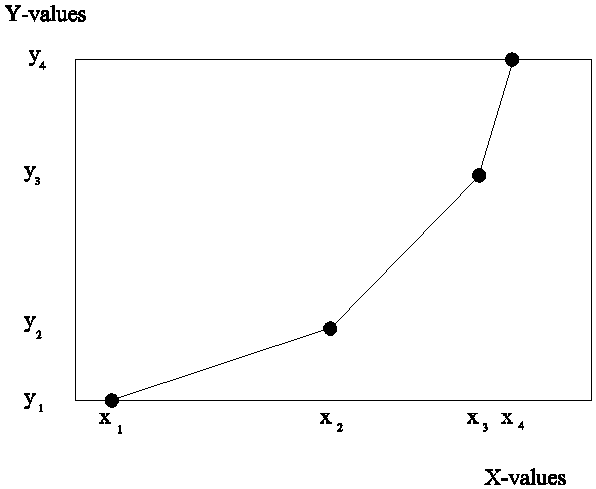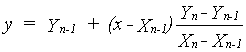## AFGEN function

AFGEN stands for Arbitrary Function GENerator (Subroutine AFGen). It is a function which is used for linear interpolation in a one-dimensional array with paired data. Uneven places in the array represent the X-values, whereas the Y-values are represented by the even places. Such an array can describe the dependency of variable Y on variable X when no mathematical description is available or is too cumbersome. A plotted example is depicted in Figure AIII_1:Figure AIII_1 Linear interpolation

The array belonging to this example has to be filled as:

 Place (1) (2) (3) (4) (5) (6) (7) (8) Value X1 Y1 X2 Y2 X3 Y3 X4 Y4

Arguments of the AFGEN function in order of their place in the argument list are: name of the table, number of pairs, X value to be interpolated. The X-values have to be arranged from low to high values and are not allowed to be interchanged. Every X-value has to precede its connected Y-value.

Three situations for interpolation can occur:

1) The argument x at which interpolation should take place is less or equal to the first X-value in the array. The Y-value is set to the first Y-value in the array.

2) The argument x value at which interpolation should take place is between the first and the last X-value in the array. The Y-value can now be found via linear interpolation. First the X values left and right from the argument x have to be detected, then the Y-value can be calculated:3) The argument x at which interpolation should take place is equal to or larger then the last X-value in the array. The Y-value is set to the last Y-value in the array.